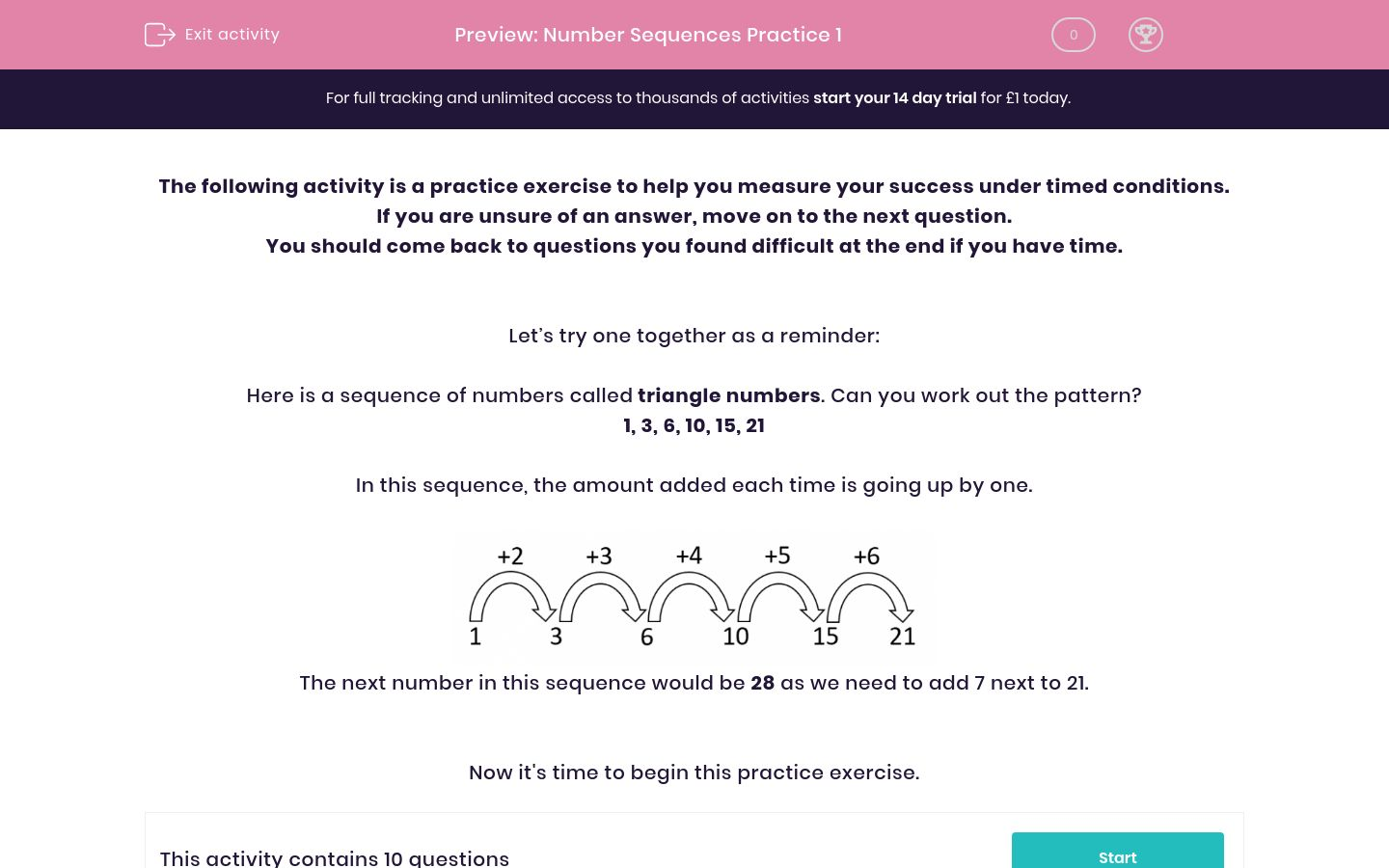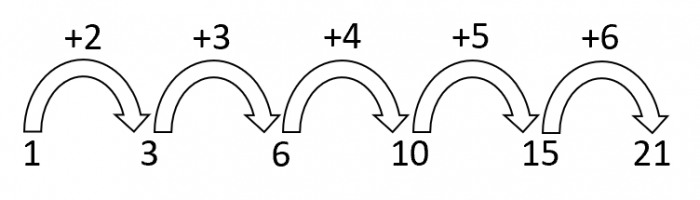Number Sequences Practice 1

In this this practice exercise, students will identify missing numbers in sequences of prime, square and triangle numbers within timed conditions.Key stage:  KS 2

Curriculum topic:  Exam-Style Questions: Verbal Reasoning

Curriculum subtopic:  Exam-Style Questions: Number Sequences

Difficulty level:QUESTION 1 of 10

The following activity is a practice exercise to help you measure your success under timed conditions.

If you are unsure of an answer, move on to the next question.

You should come back to questions you found difficult at the end if you have time.

Let’s try one together as a reminder:

Here is a sequence of numbers called triangle numbers. Can you work out the pattern?

1, 3, 6, 10, 15, 21

In this sequence, the amount added each time is going up by one.The next number in this sequence would be 28 as we need to add 7 next to 21.

Now it's time to begin this practice exercise.

Choose the number that best completes the sequence.

4, 7, 10, 13, __

18

16

17

14

19

Which number continues the pattern in this number sequence?

13, 14, 16, 19, 23, __

27

28

29

19

30

Which number continues the pattern in the sequence below?

8, 3, 16, 6, 24, 9, __

40

12

32

15

10

What number would continue the pattern below?

55, 3, 45, 4, 35, 5, __

35

16

6

25

5

Which number would continue the pattern below?

144, 121, 100, 81, __

21

64

81

25

100

Which number would come next in the following sequence?

3, 6, 10, 15, 21, __

21

26

29

27

28

Which number would come next in this sequence?

11, 13, 17, 19, __

21

29

23

20

17

Which number would come next in the following sequence?

4, 5, 9, 18, 34, __

59

44

87

50

78

Which number would come next in the following sequence?

3, 4, 6, 7, 9, __

9

10

11

12

13

Which number would come next in the following sequence?

23, 29, 31, 37, __

39

41

40

38

42

• Question 1

Choose the number that best completes the sequence.

4, 7, 10, 13, __

16
EDDIE SAYS
Did you notice that this sequence is going up in 3s? 13 + 3 = 16 Always check how much the sequence is going up or down by and whether the jumps are staying the same or changing.
• Question 2

Which number continues the pattern in this number sequence?

13, 14, 16, 19, 23, __

28
EDDIE SAYS
We have a changing number sequence here. We +1 to the first number, +2 to the second, +3 to the third and +4 to the fourth. This means that we need to +5 to get the next number in the sequence. 23 + 5 = 28• Question 3

Which number continues the pattern in the sequence below?

8, 3, 16, 6, 24, 9, __

32
EDDIE SAYS
We had alternate patterns going on here. This means that there are two patterns working at the same time - greedy!The 1st, 3rd, and 5th numbers in the sequence are going up in 8s. The 2nd, 4th and 6th numbers in the sequence are going up in 3s. To work out the 7th number, we need to carry on the sequence of 8s. 24 + 8 = 32. Always label the jumps in this type of question to help you work out the single pattern or alternate patterns.
• Question 4

What number would continue the pattern below?

55, 3, 45, 4, 35, 5, __

25
EDDIE SAYS
Did you notice that we have another set of alternate patterns going on here? We are subtracting 10 from the 1st, 3rd and 5th numbers in the sequence. We are adding 1 to the 2nd, 4th and 6th numbers in the sequence. This means that we need to subtract 10 from 35 to give us the 7th number in the sequence. This makes 25 the correct answer.• Question 5

Which number would continue the pattern below?

144, 121, 100, 81, __

64
EDDIE SAYS
All of the numbers here are square numbers. The numbers are decreasing, so we need to find the square number that comes before 81. 81 is 9 squared which means that we need to write the answer to 8 squared. 8 squared is 64.
• Question 6

Which number would come next in the following sequence?

3, 6, 10, 15, 21, __

28
EDDIE SAYS
We have a changing number sequence here. The amount we are adding changes each time. We +3 to the first number, +4 to the second, +5 to the third and +6 to the fourth. This means that we need to +7 to the fifth number. 21 + 7 = 28.Remember to label your jumps to help you keep track.
• Question 7

Which number would come next in this sequence?

11, 13, 17, 19, __

23
EDDIE SAYS
All of the numbers in this sequence are prime numbers. The next prime number after 19 is 23. Remember that a prime number only has 2 factors: 1 and itself. Which means that they won't appear in our times tables too (except in the 1 x spot).
• Question 8

Which number would come next in the following sequence?

4, 5, 9, 18, 34, __

59
EDDIE SAYS
We have another changing number sequence here, which means we are adding a different number each time. We +1 to the first number, +4 to the second, +9 to the third and +16 to the fourth. What do you notice about the numbers 1, 4, 9 and 16? They are all square numbers! This means we need to add the next square number, which is 25. 34 + 25 = 59• Question 9

Which number would come next in the following sequence?

3, 4, 6, 7, 9, __

10
EDDIE SAYS
We have a changing number sequence here. We swap between adding 1 and adding 2 in this sequence. We +2 to 7 to get 9, which means we need to +1 to 9 to get to the correct answer of 10.Carefully label all of the jumps between the numbers to help you work out the rule.
• Question 10

Which number would come next in the following sequence?

23, 29, 31, 37, __

41
EDDIE SAYS
Did you use your number detective skills to identify these as prime numbers? The next prime number after 37 is 41. It can't be 39 as that would be in the 3 times table. Excellent sequence spotting!
---- OR ----

Sign up for a £1 trial so you can track and measure your child's progress on this activity.

What is EdPlace?

We're your National Curriculum aligned online education content provider helping each child succeed in English, maths and science from year 1 to GCSE. With an EdPlace account you’ll be able to track and measure progress, helping each child achieve their best. We build confidence and attainment by personalising each child’s learning at a level that suits them.

Get startedSimilar activities you may like

Start your £1 trial today.
Subscribe from £10/month.